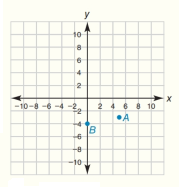Chapter 10.CT, Problem 3CTElementary Geometry For College St...

7th Edition
Alexander + 2 others
ISBN: 9781337614085

Solutions

Chapter
SectionElementary Geometry For College St...

7th Edition
Alexander + 2 others
ISBN: 9781337614085
Textbook Problem

Use d = ( x 2 - x 1 ) 2 + ( y 2 - y 1 ) 2 to find the length of C D - as described in Exercise 2.In the coordinate system for Exercise 1, plot and label each point: C ( - 6 ,   1 ) and D ( 0 ,   9 )To determine

To find:

The length of CD- using distance formula where the coordinates are C(-6, 1) and D0, 9.

Explanation

Given points are,

C(-6, 1) and D(0, 9)

Point C is located at a distance from origin in the x-axis =-6

Point C is located at a distance from origin in the y-axis =1

Similarly, point D is located at a distance from origin in the x-axis =0

Point D is located at a distance from origin in the y-axis =9

Sketch the given points in graph and name them as C and D and joined the points as shown in the figure,

Formula for distance is, d=(x2-x1)2+(y2

Still sussing out bartleby?

Check out a sample textbook solution.

See a sample solution

The Solution to Your Study Problems

Bartleby provides explanations to thousands of textbook problems written by our experts, many with advanced degrees!

Get Started

Evaluate the indefinite integral. x3x2+1dx

Single Variable Calculus: Early Transcendentals, Volume I

In Exercises 7-28, perform the indicated operations and simplify each expression. 17. 1+1x11x

Applied Calculus for the Managerial, Life, and Social Sciences: A Brief Approach

A sample with a mean of M = 8 has X = 56. How many scores are in the sample?

Essentials of Statistics for The Behavioral Sciences (MindTap Course List)